# Mathematics Commons™

20,257 Full-Text Articles 20,188 Authors 7,076,253 Downloads299 Institutions

## All Articles in Mathematics

20,257 full-text articles. Page 620 of 636.

Parallelization Of The Wolff Single-Cluster Algorithm, 2010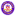University of Latvia

#### Parallelization Of The Wolff Single-Cluster Algorithm, Jevgenijs Kaupužs, Jānis Rimšāns, Roderick V.N. Melnik

##### Mathematics Faculty Publications

A parallel [open multiprocessing (OpenMP)] implementation of the Wolff single-cluster algorithm has been developed and tested for the three-dimensional (3D) Ising model. The developed procedure is generalizable to other lattice spin models and its effectiveness depends on the specific application at hand. The applicability of the developed methodology is discussed in the context of the applications, where a sophisticated shuffling scheme is used to generate pseudorandom numbers of high quality, and an iterative method is applied to find the critical temperature of the 3D Ising model with a great accuracy. For the lattice with linear size L=1024, we have ...

Frontal Reaction In A Layered Polymerizing Medium, 2010Bridgewater State University

#### Frontal Reaction In A Layered Polymerizing Medium, Dmitry Golovaty, Laura K. Gross, James T. Joyner

##### Mathematics and Computer Science Faculty Publications

We analyze the dynamics of a reaction propagating along a two-dimensional medium of nonuniform composition. We consider the context of a self-sustaining reaction front that converts a monomer-initiator mixture into an inhomogeneous polymeric material. We model the system with one-step effective kinetics, assuming large activation energy. Using asymptotic methods, we find the analytical expressions for the front profile as well as monomer and temperature distributions. Further, we demonstrate that the predictions of the asymptotic theory match well with the numerical simulations.

Fast Computation Of Kernel Estimators, 2010University of Pennsylvania

#### Fast Computation Of Kernel Estimators, Vikas C. Raykar, Ramani Duraiswami, Linda H. Zhao

##### Statistics Papers

The computational complexity of evaluating the kernel density estimate (or its derivatives) at m evaluation points given n sample points scales quadratically as O(nm)—making it prohibitively expensive for large datasets. While approximate methods like binning could speed up the computation, they lack a precise control over the accuracy of the approximation. There is no straightforward way of choosing the binning parameters a priori in order to achieve a desired approximation error. We propose a novel computationally efficient ε-exact approximation algorithm for the univariate Gaussian kernel-based density derivative estimation that reduces the computational complexity from O(nm) to ...

2010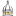University of Southern Mississippi

#### An F4-Style Involutive Basis Algorithm, Miao Yu

##### Mathematics Student Presentations

This paper introduces a new algorithm for computing Gröbner bases. To avoid as much ambiguity as possible, this algorithm combines the F4 algorithm and basic algorithm of involutive bases and it replaces the symbolic precomputation of S-polynomials and ordinary division in F4 by a new symbolic precomputation of non-multiplicative prolongations and involutive division. This innovation makes the sparse matrix of F4 in a deterministic way. As an example the Cyclic-4 problem is presented.

The Probabilistic Zeta Function, 2010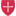College of Saint Benedict/Saint John's University

#### The Probabilistic Zeta Function, Bret Benesh

##### Mathematics Faculty Publications

This paper is a summary of results on the PG(s) function, which is the reciprocal of the probabilistic zeta function for finite groups. This function gives the probability that s randomly chosen elements generate a group G, and information about the structure of the group G is embedded in it.

Modeling Super-Spreading Events For Sars, 2010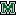Marshall University

#### Modeling Super-Spreading Events For Sars, Thembinkosi P. Mkhatshwa

##### Theses, Dissertations and Capstones

One of the intriguing characteristics of the 2003 severe acute respiratory syndrome (SARS) epidemics was the occurrence of super spreading events (SSEs). Super-spreading events for a specific infectious disease occur when infected individuals infect more than the average number of secondary cases. The understanding of these SSEs is critical to under- standing the spread of SARS. In this thesis, we present a modification of the basic SIR (Susceptible - Infected - Removed) disease model, an SIPR (Susceptible - Regular Infected - Super-spreader - Removed) model, which captures the effect of the SSEs.

2010Marshall University

#### Mathematics In Motion: Linear Systems Of Differential Equations On The Differential Analyzer, Devon A. Tivener

##### Theses, Dissertations and Capstones

In this work, I will provide an introduction to the dierential analyzer, a machine designed to solve dierential equations through a process called mechanical integration. I will give a brief historical account of dierential analyzers of the past, and discuss the Marshall University Dierential Analyzer Project. The goal of this work is to provide an analysis of solutions of systems of dierential equations using a dierential analyzer. In particular, we are interested in the points at which these systems are in equilibrium and the behavior of solutions that start away from equilibrium. After giving a description of linear systems of ...

Rayleigh Wave Dispersion Curve Inversion: Occam Versus The L1-Norm, 2010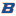Boise State University

#### Rayleigh Wave Dispersion Curve Inversion: Occam Versus The L1-Norm, Matthew M. Haney, Leming Qu

##### Mathematics Faculty Publications and Presentations

We compare inversions of Rayleigh wave dispersion curves for shear wave velocity depth profiles based on the L2-norm (Occam's Inversion) and L1-norm (TV Regularization). We forward model Rayleigh waves using a finite-element method instead of the conventional technique based on a recursion formula and root-finding. The forward modeling naturally leads to an inverse problem that is overparameterized in depth. Solving the inverse problem with Occam's Inversion gives the smoothest subsurface model that satisfies the data. However, the subsurface need not be smooth and we therefore also solve the inverse problem with TV Regularization, a procedure that does not ...

2010 Alumni Presenters, 2010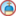University of Dayton

#### 2010 Alumni Presenters, University Of Dayton. Department Of Mathematics

##### Biennial Alumni Seminar

No abstract provided.

2010University of Dayton

#### Every Time I Turn Around There's Dr. Schraut Or You Can't Take Mathematics Out Of A U. D. Mathematics Major (Abstract), Eugene Steuerie

##### Kenneth C. Schraut Memorial Lectures

In this lecture, Gene Steurele, a math major from the 1968 graduating class, will discuss the many ways that mathematics & statistics crop up in almost every major public policy debate in which he has participated.

Eleventh Kenneth C. Schraut Memorial Lecture (Poster), 2010University of Dayton

#### Eleventh Kenneth C. Schraut Memorial Lecture (Poster), University Of Dayton. Department Of Mathematics

##### Kenneth C. Schraut Memorial Lectures

No abstract provided.

Periodic Solutions Of Neutral Delay Integral Equations Of Advanced Type, 2010University of Dayton

#### Periodic Solutions Of Neutral Delay Integral Equations Of Advanced Type, Muhammad Islam, Nasrin Sultana, James Booth

##### Mathematics Faculty Publications

We study the existence of continuous periodic solutions of a neutral delay integral equation of advanced type. In the analysis we employ three fixed point theorems: Banach, Krasnosel'skii, and Krasnosel'skii-Schaefer. Krasnosel'skii-Schaefer fixed point theorem requires an a priori bound on all solutions. We employ a Liapunov type method to obtain such bound.

Coarsening In High Order, Discrete, Ill-Posed Diffusion Equations, 2010University of Dayton

#### Coarsening In High Order, Discrete, Ill-Posed Diffusion Equations, Catherine Kublik

##### Mathematics Faculty Publications

We study the discrete version of a family of ill-posed, nonlinear diffusion equations of order 2n. The fourth order (n=2) version of these equations constitutes our main motivation, as it appears prominently in image processing and computer vision literature. It was proposed by You and Kaveh as a model for denoising images while maintaining sharp object boundaries (edges). The second order equation (n=1) corresponds to another famous model from image processing, namely Perona and Malik's anisotropic diffusion, and was studied in earlier papers. The equations studied in this paper are high order analogues of the Perona-Malik equation ...

2010University of Dayton

#### Linearly Ordered Topological Spaces And Weak Domain Representability, Joe Mashburn

##### Mathematics Faculty Publications

It is well known that domain representable spaces, that is topological spaces that are homeomorphic to the space of maximal elements of some domain, must be Baire. In this paper it is shown that every linearly ordered topological space (LOTS) is homeomorphic to an open dense subset of a weak domain representable space. This means that weak domain representable spaces need not be Baire.

Heteroclinic Solutions To An Asymptotically Autonomous Second-Order Equation, 2010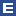Embry-Riddle Aeronautical University

#### Heteroclinic Solutions To An Asymptotically Autonomous Second-Order Equation, Gregory S. Spradlin

##### Mathematics - Daytona Beach

We study the differential equation ẍ(t) = a(t)V '(x(t)), where V is a double-well potential with minima at x = ±1 and a(t) →l > 0 as |t| → 1. It is proven that under certain additional assumptions on a, there exists a heteroclinic solution x to the differential equation with x(t) → -1 as t → -1 and x(t) → 1 as t → ∞. The assumptions allow l - a(t) to change sign for arbitrarily large values of |t|, and do not restrict the decay rate of |l -a(t)| as |t| → ∞ © 2010 Texas State University - San Marcos.

On Residual Lifetimes Of K-Out-Of-N Systems With Nonidentical Components, 2010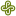Portland State University

#### On Residual Lifetimes Of K-Out-Of-N Systems With Nonidentical Components, Subhash C. Kochar, Maochao Xu

##### Mathematics and Statistics Faculty Publications and Presentations

In this article, mixture representations of survival functions of residual lifetimes of k-out-of-n systems are obtained when the components are independent but not necessarily identically distributed. Then we stochastically compare the residual lifetimes of k-out-of-n systems in one- and two-sample problems. In particular, the results extend some results in Li and Zhao , Khaledi and Shaked , Sadegh , Gurler and Bairamov  and Navarro, Balakrishnan, and Samaniego . Applications in the proportional hazard rates model are presented as well.

2010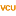Virginia Commonwealth University

#### Analysis Of The Consistency Of A Mixed Integer Programming-Based Multi-Category Constrained Discriminant Model, J. Paul Brooks, Eva K. Lee

##### Statistical Sciences and Operations Research Publications

Classification is concerned with the development of rules for the allocation of observations to groups, and is a fundamental problem in machine learning. Much of previous work on classification models investigates two-group discrimination. Multi-category classification is less-often considered due to the tendency of generalizations of two-group models to produce misclassification rates that are higher than desirable. Indeed, producing “good” two-group classification rules is a challenging task for some applications, and producing good multi-category rules is generally more difficult. Additionally, even when the “optimal” classification rule is known, inter-group misclassification rates may be higher than tolerable for a given classification model ...

Curvature Measures For Nonlinear Regression Models Using Continuous Designs With Applications To Optimal Design, 2010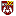Loyola University Chicago

#### Curvature Measures For Nonlinear Regression Models Using Continuous Designs With Applications To Optimal Design, Timothy O'Brien, Somsri Jamroenpinyo, Chinnaphong Bumrungsup

##### Mathematics and Statistics: Faculty Publications and Other Works

We present and illustrate the methodology to calculate curvature measures for continuous designs, and extend design criteria to incorporate continuous designs. These design algorithms include quadratic design procedures, a subset design criterion, a second-order mean-square error design criterion, and a marginal curvature design methodology. A discussion of confidence intervals is also provided for continuous designs.

Zeros Of Some Level 2 Eisenstein Series, 2010Western Washington University

#### Zeros Of Some Level 2 Eisenstein Series, Sharon Garthwaite, Ling Long, Holly Swisher, Stephanie Treneer

##### Mathematics

The zeros of classical Eisenstein series satisfy many intriguing properties. Work of F. Rankin and Swinnerton-Dyer pinpoints their location to a certain arc of the fundamental domain, and recent work by Nozaki explores their interlacing property. In this paper we extend these distribution properties to a particular family of Eisenstein series on Γ(2) because of its elegant connection to a classical Jacobi elliptic function cn(u) which satisfies a differential equation (see formula (1.2)). As part of this study we recursively define a sequence of polynomials from the differential equation mentioned above that allow us to calculate zeros ...

Signature Matrices: The Eigenvalue Problem, 2010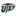University of Texas at El Paso

#### Signature Matrices: The Eigenvalue Problem, Valeria Aguirre Holguin

##### Open Access Theses & Dissertations

Dealing with matrices can give us a hard time, especially when their dimension is too big, but we also well know how valuable information a matrix may carry, and that is why we study them. When a matrix has a significant number of zeroes we realize how much easier all calculations are. For instance, the product will be simpler to calculate, the determinant, the inverse and even the eigenvalue problem. This thesis provides the description and behavior of a very special kind of matrices which we call signature matrices. A particular feature of these matrices lies in the fact that ...